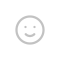## 均衡器（Equalizer）

$H(jw)=\frac{1}{1+\frac{\omega}{\omega_{c}}}$

$\theta(\omega)=-arctan(\frac{\omega}{\omega_{c}})$

$H(j\omega) = \frac{ j\omega – \omega_c }{ j\omega + \omega_c }$

$\theta(\omega)=-2arctan(\frac{\omega}{\omega_{c}})$

## 延迟器（Delay）

$Y[t]=X[t]+a*Y[t-T]$

## 混响器（Reverb）图片来源：Auditory Room Size Perception for Real Rooms， Claudiu B. Pop & Densil Cabrera来源：”architectural acoustics”, W. Cavanaugh, J. A. Wilkes, version 1999, p. 259

$(f*g)(n)=\sum_{\tau=-\infty }^{\infty}f(\tau)g(n-\tau)$

https://www.zhihu.com/question/22298352

## 发送评论编辑评论Emoji